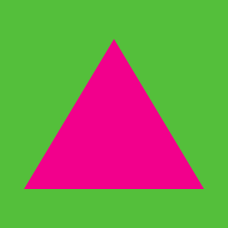Geometry

# Properties of Triangles: Level 3 Challenges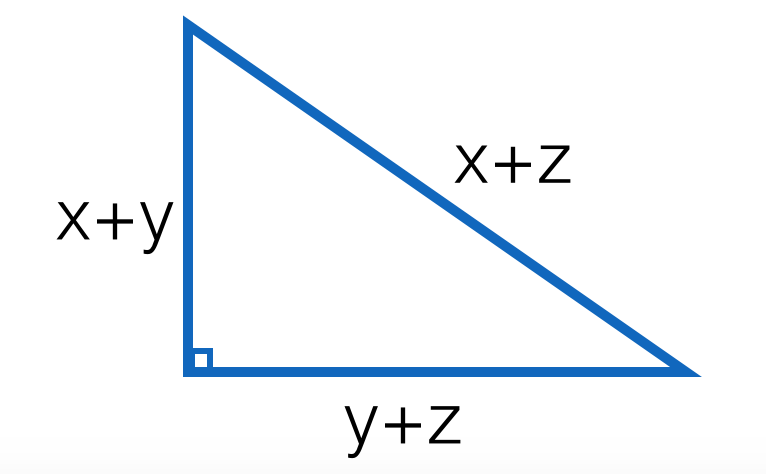In a certain right triangle, the hypotenuse equals $(x+z)$ while the other two sides are $(x+y)$ and $(y+z)$ for positive integers $x, y, z$.

If $y+4=z$ and $z>x$ , then compute $\dfrac{z}{x}$.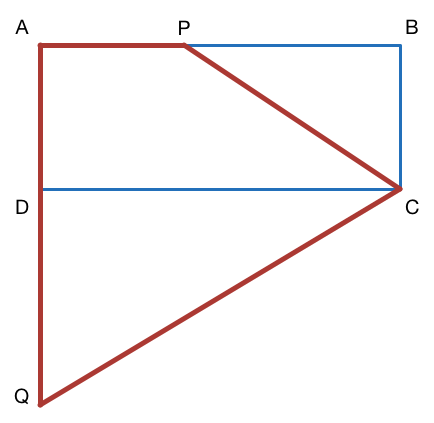Consider a rectangle which has a diagonal of length 6. If $P$ is a point on side $AB$ such that $\lvert\overline{AP}\rvert=\lvert\overline{AD}\rvert,$ and $Q$ is a point on the extension of side $AD$ such that $\lvert\overline{AQ}\rvert=\lvert\overline{AB}\rvert,$ what is the area of the quadrilateral $APCQ?$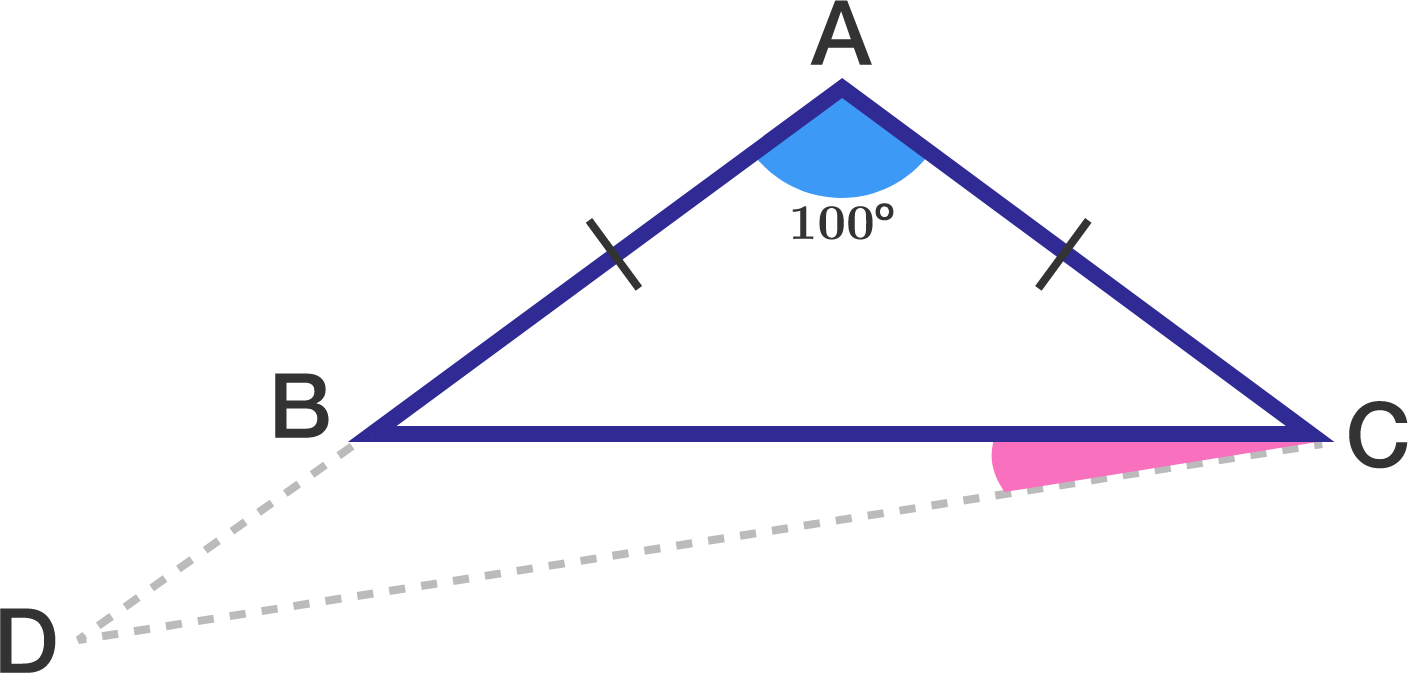In triangle $ABC,$ $AB = AC$ and $\angle BAC = 100^\circ.$ If $\overline{AB}$ is extended to $D$ such that $AD=BC,$ find $\angle BCD$ (in degrees).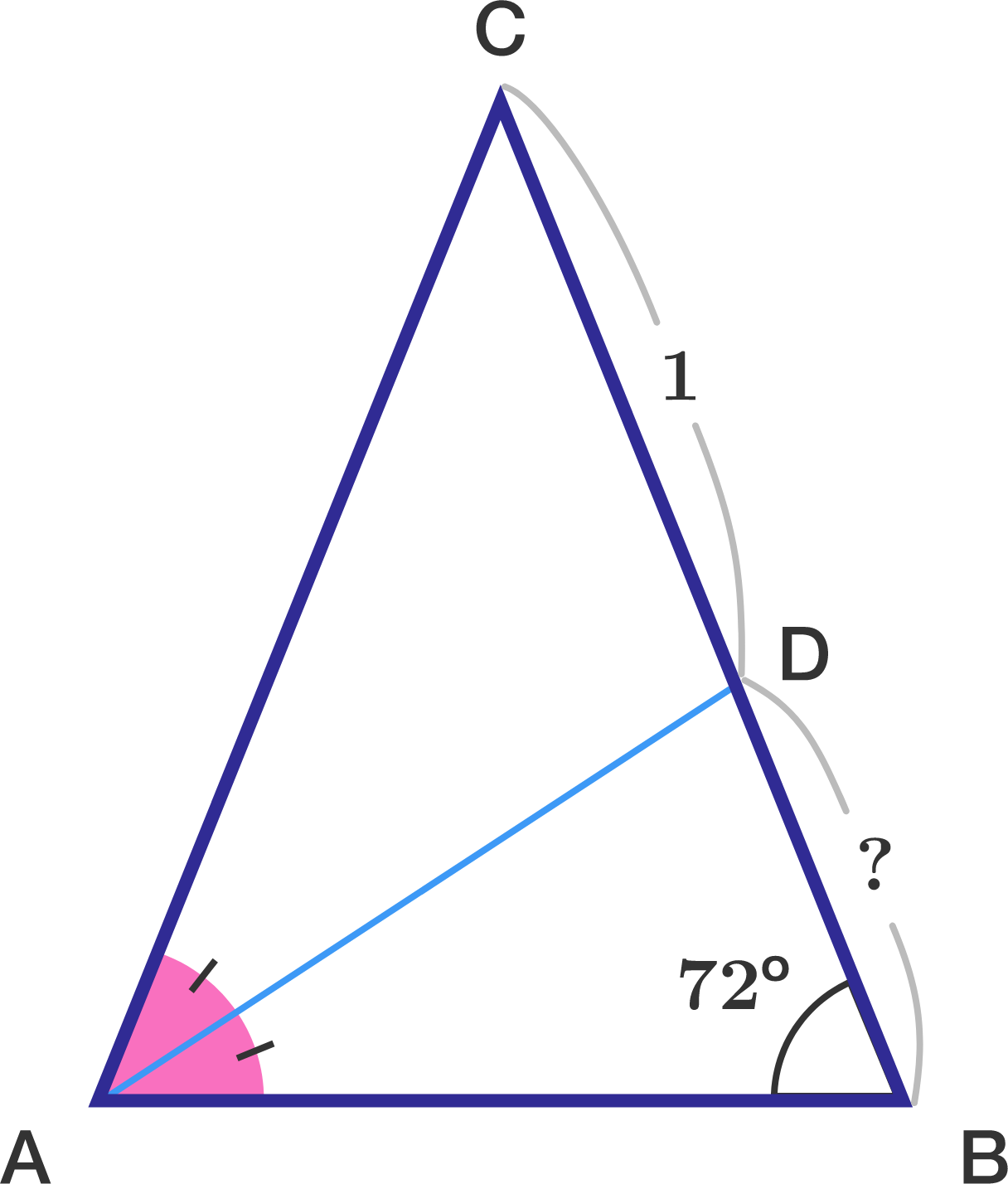$ABC$ is an isosceles triangle with $AC = BC$. Furthermore, $D$ is a point on $BC$ that bisects the angle at $A$.

If $\angle B = 72^\circ$ and $CD=1,$ then find length of $BD$ (upto 3 decimal places).

In a triangle with integer side lengths, one side is three times as long as a second side, and the length of the third side is 17. What is the greatest possible perimeter of the triangle?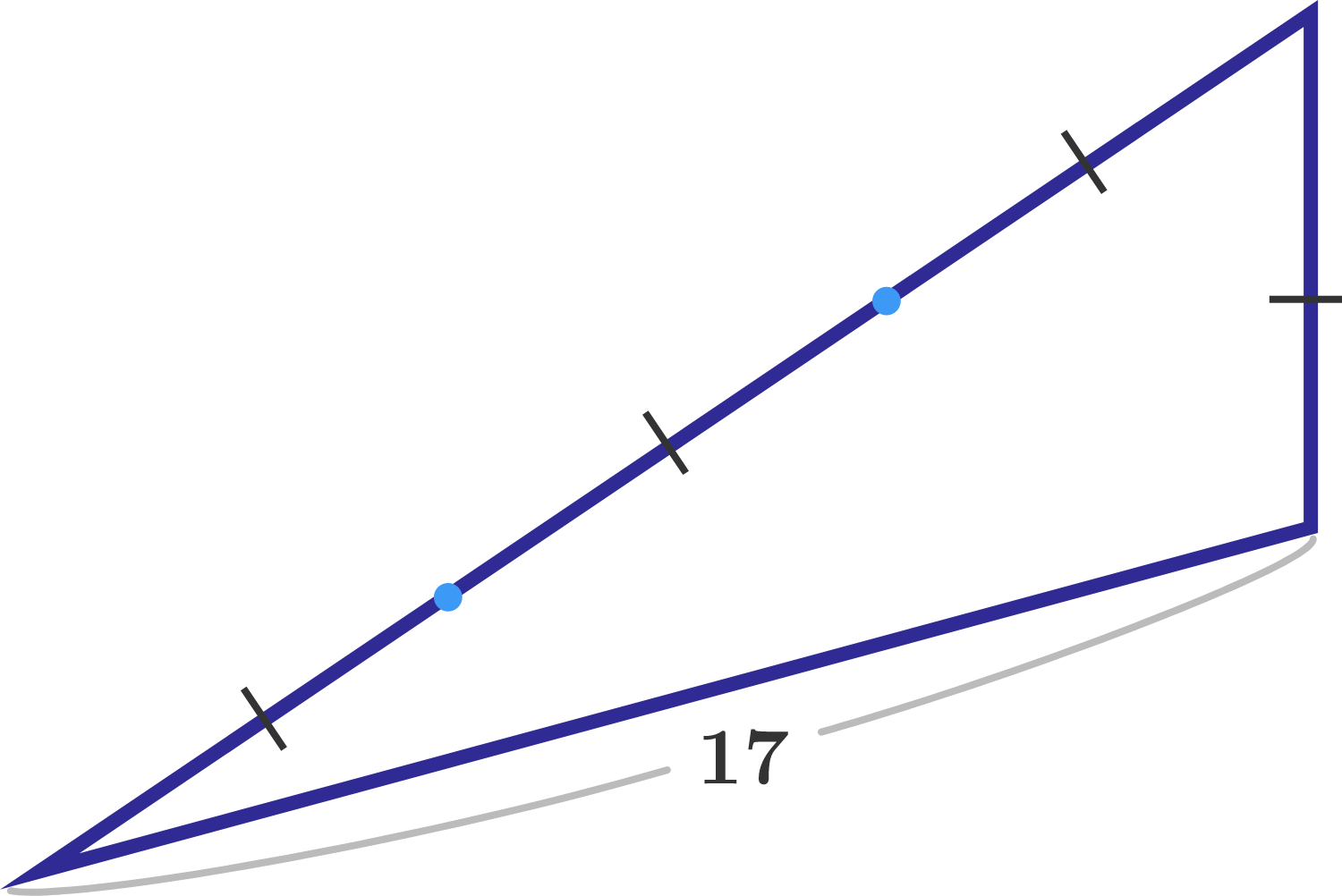×